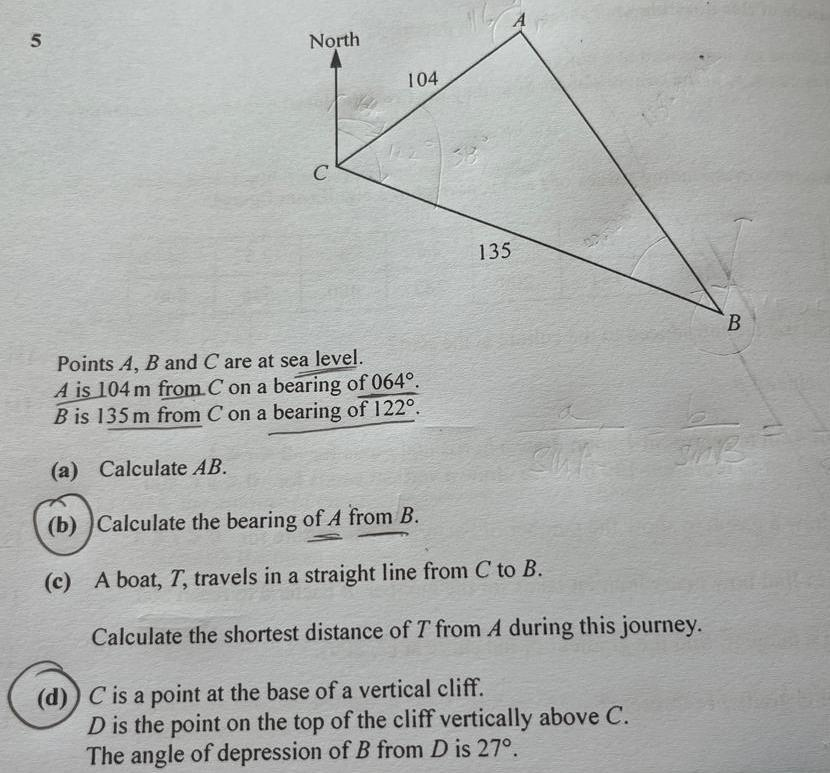Question:

# 5 North C 104 33 135 Points A B and C are at sea level A is

Last updated: 8/17/20235 North C 104 33 135 Points A B and C are at sea level A is 104 m from C on a bearing of 064 Bis 135 m from C on a bearing of 122 a Calculate AB b Calculate the bearing of A from B c A boat T travels in a straight line from C to B Calculate the shortest distance of T from A during this journey d C is a point at the base of a vertical cliff D is the point on the top of the cliff vertically above C The angle of depression of B from D is 27 B